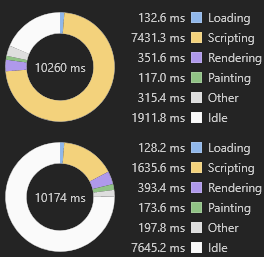## break_infinity.jsA replacement for https://github.com/MikeMcl/decimal.js/ for incremental games which need to deal with very large numbers (bigger in magnitude than 1e308, up to as much as 1e9e15) and want to prioritize speed over accuracy.

If you want to prioritize accuracy over speed, please use decimal.js instead.

NEW:

You can use break_infinity.js directly from a CDN via a script tag:

``````    <!-- You can load it as a minified file (recommended) -->    <script src="https://cdn.jsdelivr.net/npm/break_infinity.js@2"></script>    <!-- ...or as a non-minified file (for debugging) -->    <script src="https://cdn.jsdelivr.net/npm/break_infinity.js@2/dist/break_infinity.js"></script>
``````

or as a JS module using import

``````    import Decimal from "break_infinity.js";
``````

or as a Node.js module using require.

``````    var Decimal = require("break_infinity.js");
``````

For the module approaches, the library is available from the npm registry

``````    \$ npm install --save break_infinity.js
``````

If you are already using decimal.js, just swap out for break_infinity.js and everything will work the same (if there's a missing function or behavioural difference, open an issue and I'll take a look).

## Use

The library exports a single class Decimal, constructor of which accepts a `Number`, `String` or `Decimal`.

``````    const x = new Decimal(123.4567);    const y = new Decimal("123456.7e-3");    const z = new Decimal(x);    const equals = x.equals(y) && y.equals(z) && x.equals(z); // true
``````

The methods that return a Decimal can be chained.

``````    const short = x.dividedBy(y).plus(z).times(9).floor();    const long = x.times("1.23456780123456789e+9")      .plus(9876.5432321)      .dividedBy("4444562598.111772")      .ceil();
``````

For the complete list of functions refer to API docs, decimal.js docs or check out Typescript definitions

## Benchmarks

So how much faster than decimal.js is break_infinity.js? Operations per second comparison using the same computer with these benchmarks link link:

Project decimal.js break_infinity.js Speedup
`new Decimal("1.23456789e987654321")` 1.6e6 4.5e6 2.8x
`Decimal.add("1e999", "9e998")` 1.3e6 3.2e6 2.5x
`Decimal.mul("1e999", "9e998")` 1.3e6 3.8e6 2.9x
`Decimal.log10("987.654e789")` 3.9e4 4.7e6 121x
`Decimal.exp(1e10)` 1.1e4 4.3e6 401x
`Decimal.pow(987.789, 123.321)` 1.3e4 5.8e6 442x

Antimatter Dimensions script time improved by 4.5x after swapping from decimal.js to break_infinity.js. This could be your incremental game:## Build

First, clone the repo

``````git clone git://github.com/Patashu/break_infinity.js.gitcd break_infinity.js
``````

Then install npm dependencies

``````npm ci
``````

And then run build command which will build all targets to the dist directory.

``````npm run build
``````

## Acknowledgements

Dedicated to Hevipelle, and all the CPUs that struggled to run Antimatter Dimensions.

Special thanks to projects from which I have sourced code or ideas from:

Additional thanks to https://github.com/Razenpok for porting the code to TypeScript and cleaning up this README.

## Other Number Libraries

Generated using TypeDoc